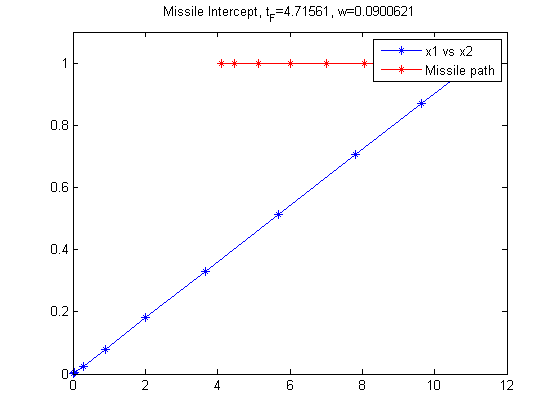68  Missile Intercept

Egwald Mathematics: Optimal Control, Intercept Missile, Elmer G. Wiens

68.1  Problem Description

Find scalar w over t in [0; t_F ] to minimize:

 J = 0

subject to:

 dx1 dt
= x3
 dx2 dt
= x4
 dx3 dt
= cos(w
 dx4 dt
= sin(w
 x(t0) = [0  0  0  0]
 x(tf) = [4+1.5*tf  1 ]
0 <= w <=
 pi 4

Reference: 

68.2  Problem setup

toms t t_f w
p = tomPhase('p', t, 0, t_f, 10);
setPhase(p);

tomStates x1 x2 x3 x4

% Initial guess
x0 = {t_f == 10; w == 0.2};

% Box constraints
cbox = {1 <= t_f <= 1e4
0 <= w  <= pi/4};

% Boundary constraints
cbnd = {initial({x1 == 0; x2 == 0
x3 == 0; x4 == 0})
final({x1 == 4+1.5*t_f; x2 == 1})};

% ODEs and path constraints
ceq = collocate({dot(x1) == x3; dot(x2) == x4
dot(x3) == cos(w); dot(x4) == sin(w)});

% Objective
objective = 0;

68.3  Solve the problem

options = struct;
options.name = 'Missile Intercept';
solution = ezsolve(objective, {cbox, cbnd, ceq}, x0, options);
t  = subs(collocate(t),solution);
x1 = subs(collocate(x1),solution);
x2 = subs(collocate(x2),solution);
w  = subs(w,solution);
t_f = subs(t_f,solution);
Problem type appears to be: lpcon
Starting numeric solver
===== * * * =================================================================== * * *
TOMLAB - Tomlab Optimization Inc. Development license  999001. Valid to 2011-02-05
=====================================================================================
Problem: ---  1: Missile Intercept              f_k       0.000000000000000000
sum(|constr|)      0.000000010885019417
f(x_k) + sum(|constr|)      0.000000010885019417
f(x_0)      0.000000000000000000

Solver: snopt.  EXIT=0.  INFORM=1.
SNOPT 7.2-5 NLP code
Optimality conditions satisfied

FuncEv    1 ConstrEv   13 ConJacEv   13 Iter   10 MinorIter   43
CPU time: 0.031250 sec. Elapsed time: 0.031000 sec.

68.4  Plot result

figure(1);
plot(x1,x2,'*-');
hold on
plot(4+1.5*t,ones(length(t)),'-*r');
legend('x1 vs x2','Missile path');
title(sprintf('Missile Intercept, t_F=%g, w=%g',t_f,w));
ylim([0 1.1]);